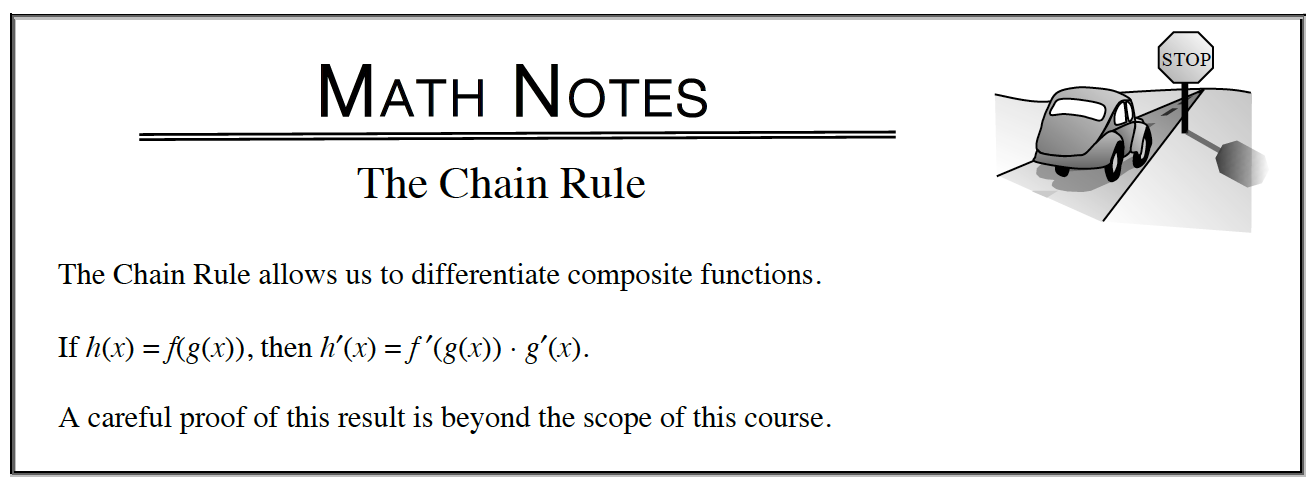Home > APCALC > Chapter 7 > Lesson 7.1.5 > Problem7-46

7-46.

Differentiate each of the following functions. Homework Help ✎

1. $y=2^{3x^2−x}+6x^{−0.4}$

Chain Rule

2. $y=6x\ln(−3y)−x^{6^2}$

Implicit differentiation.

3. $y = \frac{3}{7}\cos(11^{0.2x})$

Chain Rule